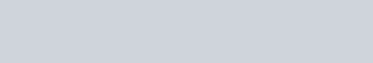Potassium permanganate on heating at 513 K gives a product which is :
Question:

Potassium permanganate on heating at 513 K gives a product which is :

1.  paramagnetic and colourless

2.  diamagnetic and green

3. diamagnetic and colourless

4. paramagnetic and green

Correct Option: , 4

Solution:In $\mathrm{K}_{2} \mathrm{Mn} \mathrm{O}_{4}$, manganese oxidation state is $+6$ and

hence it has one unpaired $\mathrm{e}^{-} .$